Levels »

# I’d Rather Be Surfing

### The Pit, Elevation: -19.2m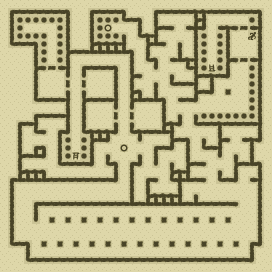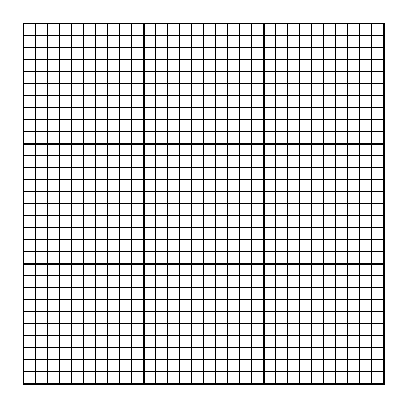### Items

•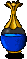Clear Blue Potion (17, 25)
•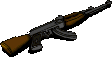AK-47 Assault Rifle (30, 3) (on Pedro)

•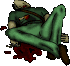(30, 3) Pedro
•AK-47 Assault Rifle

### Monsters

•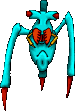44 Skitters (Random rate: 6) (12, 2) - (13, 2) - (14, 2) - (21, 2) - (21, 3) - (22, 3) - (29, 4) - (18, 5) - (28, 5) - (30, 5) - (6, 8) - (10, 8) - (5, 10) - (6, 10) - (10, 10) - (11, 10) - (22, 10) - (11, 12) - (12, 12) - (16, 12) - (5, 14) - (12, 14) - (16, 14) - (17, 14) - (25, 16) - (28, 16) - (21, 17) - (2, 19) - (21, 19) - (28, 22) - (30, 24) - (6, 25) - (10, 25) - (15, 25) - (23, 25) - (29, 25) - (16, 26) - (7, 29) - (8, 30) - (15, 30) - (16, 30) - (17, 30) - (23, 30) - (27, 30)
•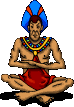3 Sentinels (16, 5) - (14, 9) - (8, 14)
•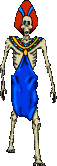13 Ghasts (Random rate: 2) (12, 2) - (28, 3) - (29, 3) - (5, 8) - (11, 8) - (17, 12) - (11, 14) - (12, 22) - (12, 23) - (16, 23) - (18, 27) - (9, 28) - (28, 28)

### Locations

• Suffocation room trigger (29, 5)
• 4 Door openers (5, 6) - (29, 7) - (8, 9) - (14, 13)
• 2 South-facing Sentinel barriers (16, 5) - (8, 14)
• North-facing Sentinel barrier (14, 9)
• 2 Save runes(12, 2) - (14, 17)